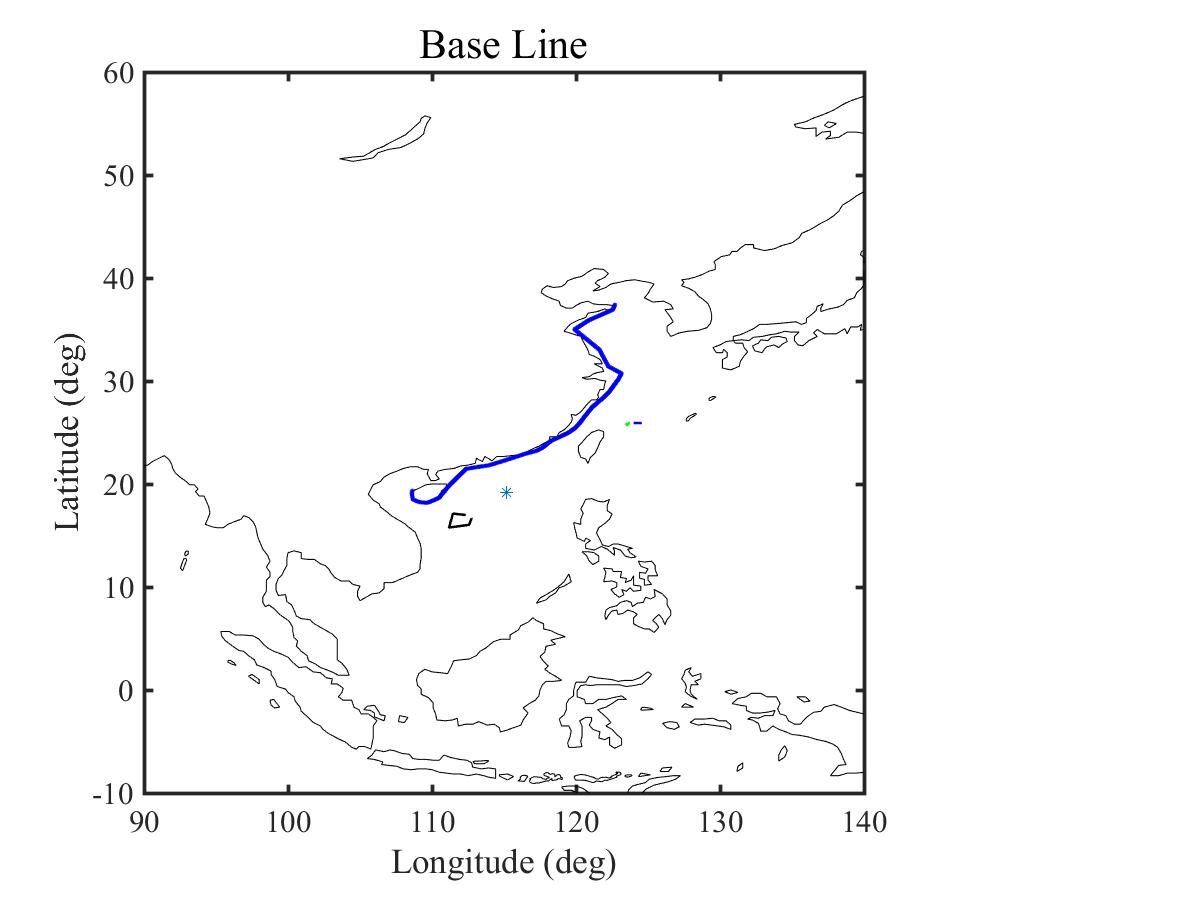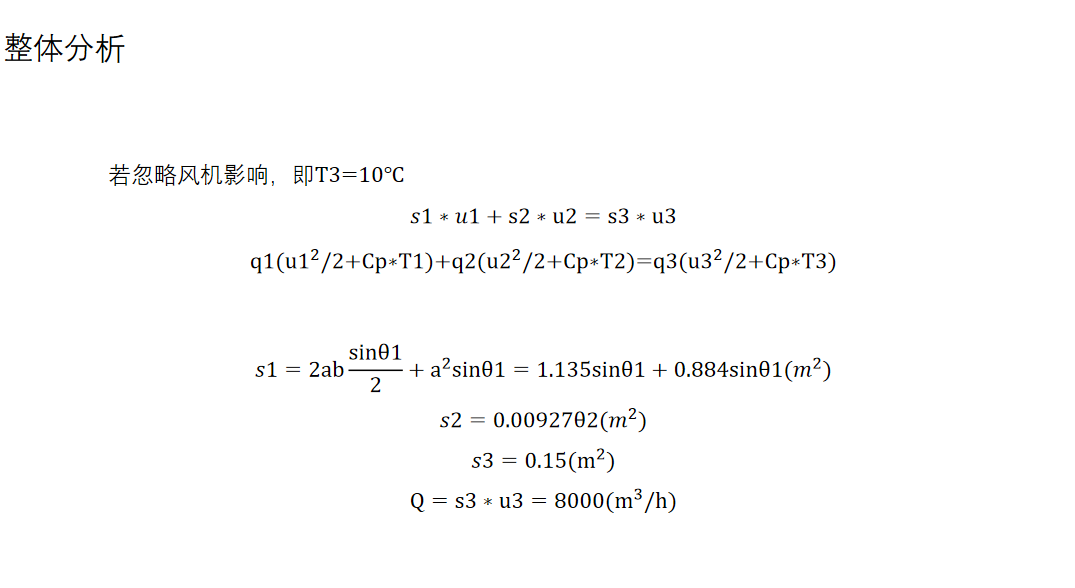###### 也许你在飞

2019-04-25 10:49 阅读 4.4k

# 请问各位大佬，怎么在MATLAB中怎么计算2个经纬度之间的距离呀？• 点赞
• 写回答
• 关注问题
• 收藏
• 复制链接分享

#### 4条回答默认 最新

• 已采纳

根据数学公式：弧长=弧度乘以半径
所以你可help以下distance这个函数，得到两个点之间的夹角
dist = distance(lat1,lon1,lat2,lon2)；
其中
lat1，lon1为第一个点的纬度和经度，单位为角度
lat2，lon2为第二个点的纬度和经度，单位为角度
返回结果为两点的夹角，转化为弧度，
dist2=dist/180 * pi
然后根据公公式
两点球面距离（弧长）=dist2（弧度） * 地球半径（6370）

特别提醒下：经纬度坐标是分种类的，具体有百度坐标系、火星坐标系、国际坐标系等，你要去研究下你的经纬度究竟是哪个的，不然容易产生误差哟。

点赞 2 评论 复制链接分享
• ``````

``````
点赞 1 评论 复制链接分享
•点赞 评论 复制链接分享
• 使用Matlab软件，

• 要求：在Matlab中敲入两个温度T1，T2数值，输出两个进风口的角度θ
1和θ2；
• 它们之间满足函数关系：s1∗u1+s2∗u2=s3∗u3
q1(u1^2/2+Cp∗T1)+q2(u2^2/2+Cp∗T2)=q3(u3^2/2+Cp∗T3)

s1=2absinθ1/2+a^2sinθ1=1.135sinθ1+0.884sinθ1(m^2)
s2=0.00927θ2(m^2)
s3=0.15(m^2)
Q=s3∗u3=8000(m^3/h)
• Q的8000要转换单位成m^3/s,因为上面的都是以s来当
点赞 评论 复制链接分享